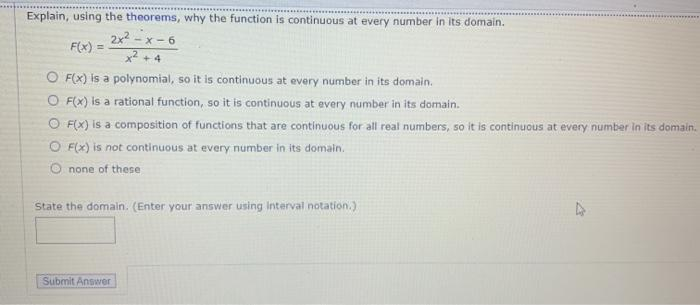### Create an Account

Home / Questions / Explain, using the theorems, why the function is continuous at every number in its domain....

# Explain, using the theorems, why the function is continuous at every number in its domain. 2x² - x-6 F(x) = +4 O F(X) is a polynomial, so it is continuous at every number in its domain. O F(x) is a ra

Explain, using the theorems, why the function is continuous at every number in its domain. 2x² - x-6 F(x) = +4 O F(X) is a polynomial, so it is continuous at every number in its domain. O F(x) is a rational function, so it is continuous at every number in its domain. O F(X) is a composition of functions that are continuous for all real numbers, so it is continuous at every number in its domain. OF(x) is not continuous at every number in its domain O none of these State the domain (Enter your answer using interval notation) Submit AnswerJun 06 2021 View more View LessSubscribe To Get Solution